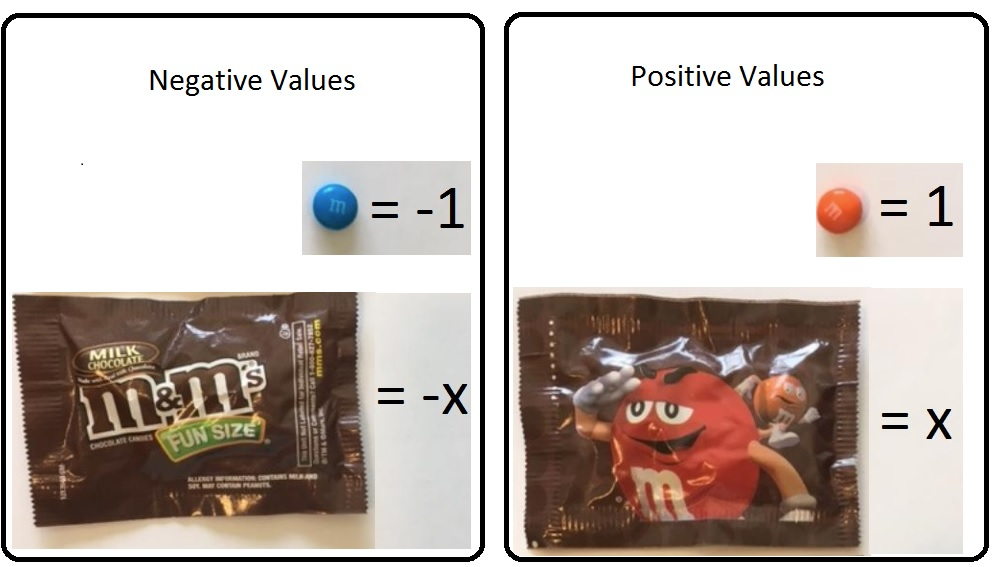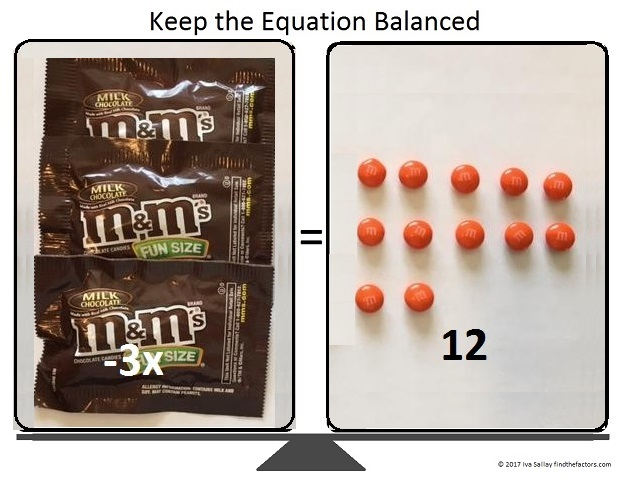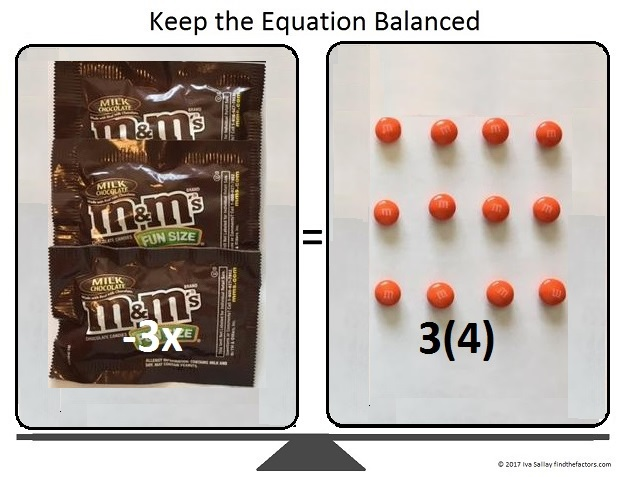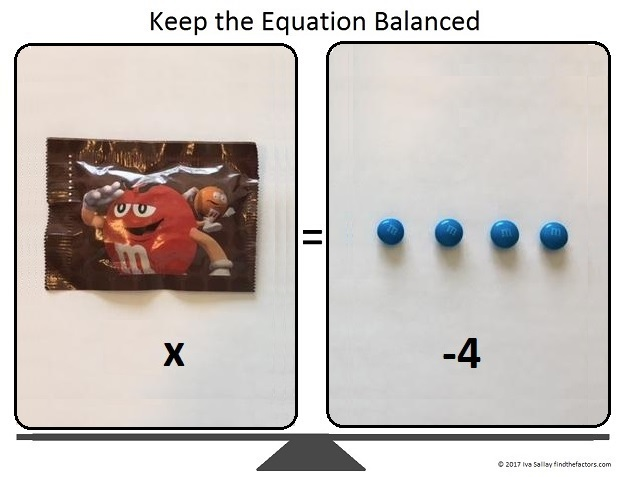# 917 How to Solve for X with Candy

How many m&m’s are there in one fun size Halloween pack of m&m’s?

I don’t know. You don’t know. Nobody knows. That’s why these little fun size packs make the perfect UNKNOWN. For this activity, there may even be a negative number of m&m’s in a pack because I’m only using blue and orange m&m’s, and I’m letting each blue m&m equal negative one and each orange m&m equal positive one.I’m also letting the front side of the fun size package equal negative x, and the back side equal positive x. In algebra we often call our unknown x.

(The colors chosen don’t matter, as long as there are only two of them, and you are consistent with that color being positive or negative. The front of the package could just as easily be +1 and the back -1. Consistency is important. Choose the values you want to use and stick with them. You can also use ALL the m&m’s in a few single packs and have the side of the candy with the m be positive and the side without the m be negative. You can use the empty wrappers as x and -x.)

We can figure out how many m&m’s are in the pack by balancing an equation. The number of m&m’s in a pack is x. We will solve for x by using the very best algebra tiles in the world, m&m’s!

Besides fun size m&m’s (or Skittles or Reece’s Pieces) we need a paper balance for our equations:Click Equation Balance for a printable pdf of the paper balance.

Now let’s solve x – 3 = 5 by using the m&m’s to find x. This is how the equation balance should look to begin:We want to get the wrapper by itself, so what do we do? To keep the equation balanced, we add three (positive) orange m&m’s (one at a time) to both sides of the paper balance:Three (negative) blue m&m’s plus three (positive) orange m&m’s are equal to zero, so we can remove them.Mmm. I just ate zero m&m’s, and they tasted so good! That leaves us with x = 8, so we have found x, and the equation has been solved! (If you have more equations to solve, you might want to wait to eat the m&m’s until you’re just about finished.)

Now let’s try finding x when the equation is a little more complicated, -2x = x + 12. This is how the balance should look at the beginning:We want to get all the x’s on one side so we subtract x from both sides of the equation by adding a (negative) front-facing wrapper to both sides of the balance.Since x – x = 0, we can remove the front-facing and back-facing wrappers from the right side of the equation:We can arrange the 12 candies into 3 rows of 4.Now we can divide both sides of the equation by 3.All that’s left to do is change the signs of EVERYTHING on both sides of the equation:Thus x = -4. We solved for x correctly because we kept the equation balanced every step of the way.

Now let me tell you a little bit about the number 917:

917 is the sum of five consecutive prime numbers:
173 + 179 + 181 + 191 + 193 = 917

Rearrange its digits and 917 becomes 197 in BASE 26.

• 917 is a composite number.
• Prime factorization: 917 = 7 × 131
• The exponents in the prime factorization are 1 and 1. Adding one to each and multiplying we get (1 + 1)(1 + 1) = 2 × 2 = 4. Therefore 917 has exactly 4 factors.
• Factors of 917: 1, 7, 131, 917
• Factor pairs: 917 = 1 × 917 or 7 × 131
• 917 has no square factors that allow its square root to be simplified. √917 ≈ 30.282007859